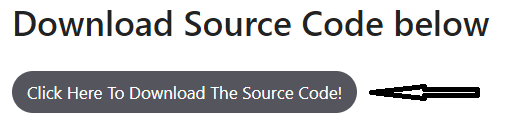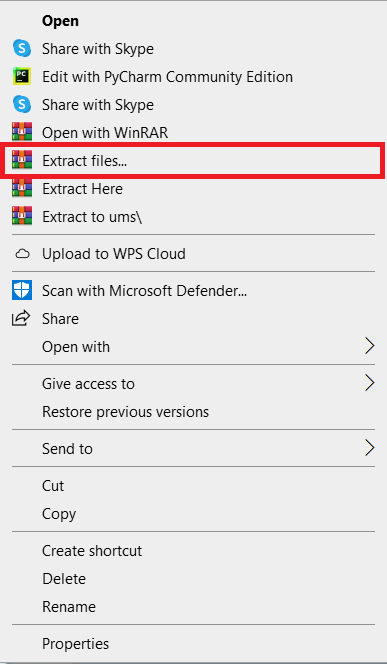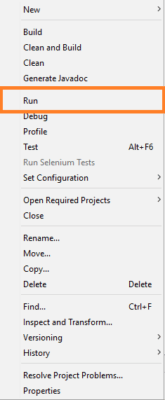# Perfect Number In Java With Source Code

The Perfect Number In Java is a fully functional console based project written in the Java programming language that works perfectly.

This Addition Of Two Numbers In Java Using Method is a fundamental project for IT students and other computer-related students. It will assist students in completing college projects and requirements. In this section, we’ll learn what a perfect number is in Java and also make Java programs. to see if the number given is perfect. We will also make a Java program to find all the perfect numbers within a certain range. The perfect number program comes up often on Java coding tests and in the classroom.

This Program For Perfect Number In Java is beneficial for practicing Java development and gaining new skills. This project is quite useful, and the concept and logic are simple to grasp. The source code is open source and is free to use. Simply scroll down and click the download button.

## What Is Perfect Number In Java

Tic Tac Toe in Java with Source Cod...
Tic Tac Toe in Java with Source Code 2021 | Java Project Source Code Free Download

The perfect number is one whose sum of factors (except the number itself) is equal to the number. In other terms, a perfect number is one whose sum of positive divisors (excluding the number itself) equals the number itself. Let’s look at an example to better comprehend it.

## Example Of A Perfect Number

Take the number 496 for example, and consider if it is a perfect number or not.

To begin, we must determine the factors of 496, which are 1, 2, 4, 8, 16, 31, 62, 124, and 248. Let’s see how many factors there are (1 + 2 + 4 + 8 + 16 + 31 + 62 + 124 + 248 = 496). The total of factors equals the number itself, as we can see. As a result, 496 is a perfect number. We may also check other numbers in the same way.

## How To Find Perfect Number In Java

1. A number should be read or initialized (n).
2. Create a variable (s) to store the value of sum.
3. Using a for/while loop, get the factors of the given integer (n).
4. Calculate the total of the factors and save the result in the variable s.
5. Compare and contrast the sum (s) and the number (n):
• The given number is a perfect number if both (s and n) are equal.
• Otherwise, the number isn’t a perfect one.

Let’s put the following steps into action in a Java program.

## Perfect Number In Java Program

```package perfectnumber_in_java;

import java.util.Scanner;

public class PerfectNumber_in_Java {

/**
* @param args the command line arguments
*/
public static void main(String[] args) {
long n, sum = 0;
Scanner sc = new Scanner(System.in);
System.out.println("Enter a number");
n = sc.nextLong();
int i = 1;
while (i <= n / 2) {
if (n % i == 0) {
sum += i;
}
i++;
}
if (sum == n) {
System.out.println(n + " is a perfect number");
} else {
System.out.println(n + " is not a  perfect number");
}
}
}
```

## Perfect Number In Java Using While Loop Project

Output 1 : Perfect Number

Output 2 : Not A Perfect Number

Anyway, if you want to level up your programming knowledge, especially Java, try this new article I’ve made for you Best Java Projects With Source Code For Beginners Free Download 2021.

To start executing this project, makes sure that you have NetBeans IDE or any platform of Java installed in your computer.

## About the Project : Perfect Number In Java With Source Code

The Perfect Number In Java was made using Java programming and the NetBeans IDE (Integrated Development Environment), but you can use any code editor as long as the project can run. This Perfect Number Logic In Java has all of the important and necessary parts that first-year, second-year, and third-year IT students can use for college projects. The whole project is written in Java, and the NetBeans code editor was used to put it together. I hope you found this article helpful as you learn to be a Java Developer.

## Perfect Number In Java : Steps On How To Run The Project

Time needed: 5 minutes.

These are the steps on how to run Perfect Number In Java• Step 2: Extract file.

Second, after you finished download the source code, extract the zip file.• Step 3: Click open project.• Step 4: Run the project.

Last, right click the project folder and click run.